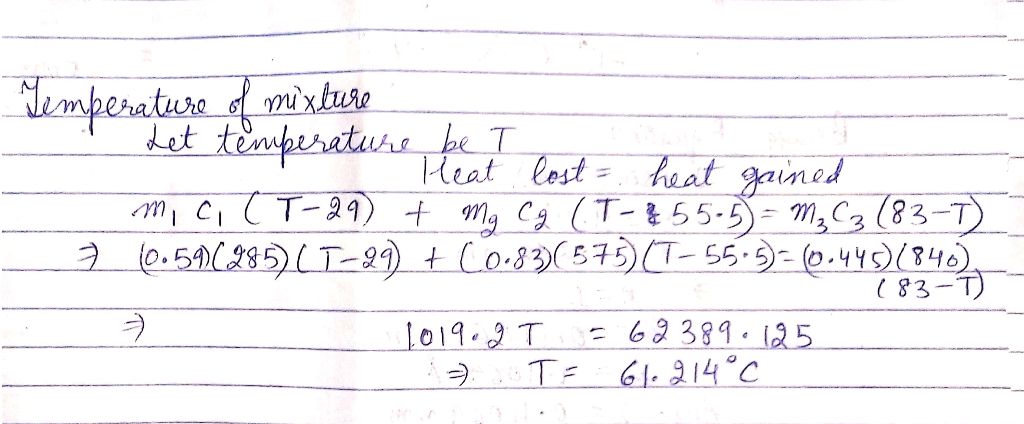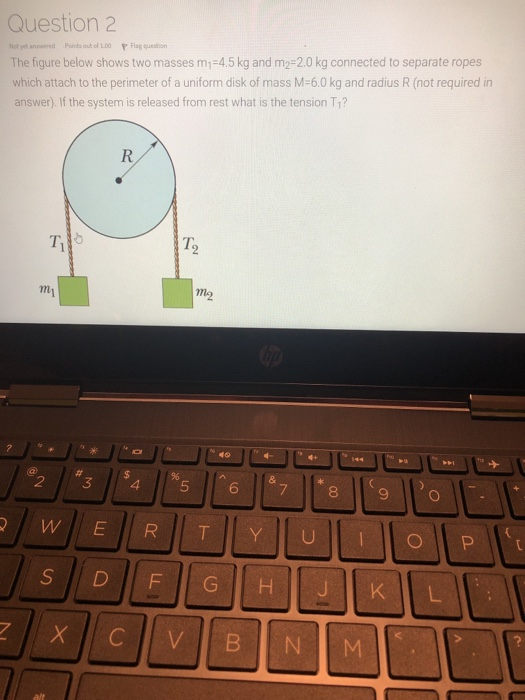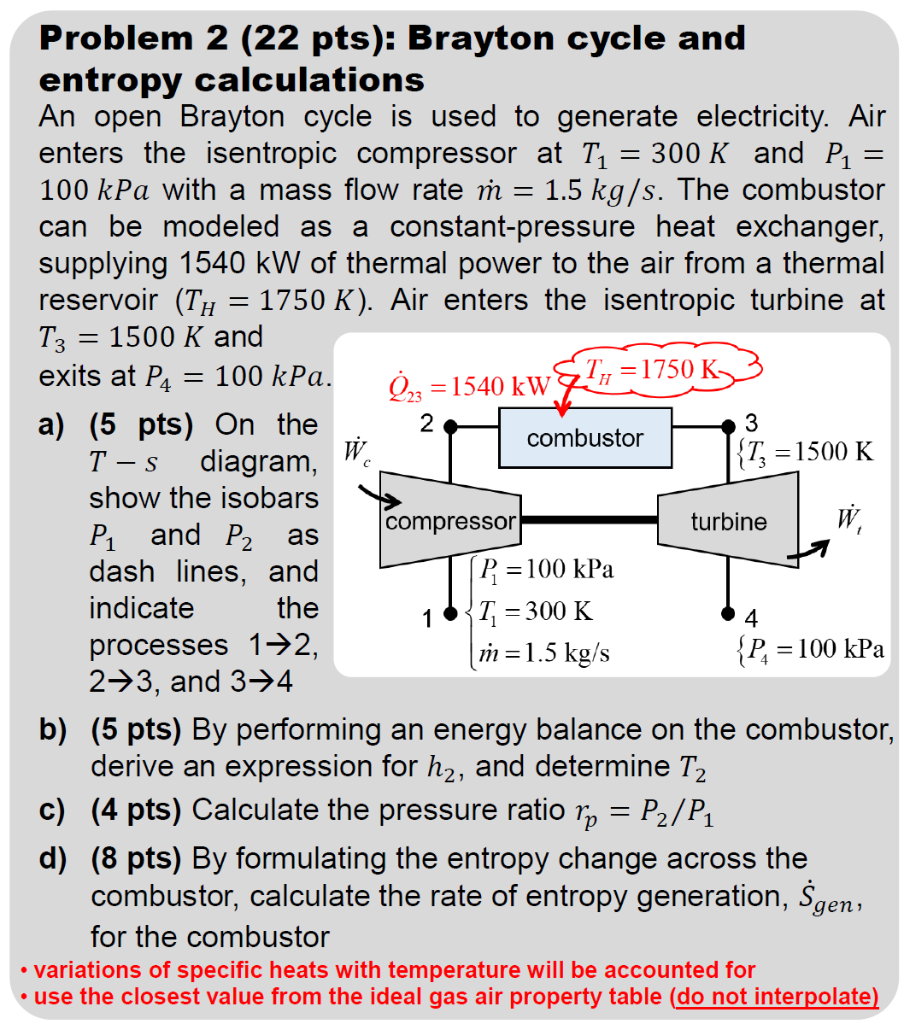Question

# Three different liquids are mixed together in a calorimeter. The masses of the liquids are: m1...

Three different liquids are mixed together in a calorimeter. The masses of the liquids are:
m1 = 590 g,
m2 = 830 g,
m3 = 445 g.
The specific heats are:
c1 = 285 J/kg°C,
c2 = 575 J/kg°C,
c3 = 840 J/kg°C.
The initial temperatures of the liquids are:
T1 = 29.0 °C,
T2 = 55.5 °C,
T3 = 83.0 °C.
What will be the temperature of the mixture in Celsius? (Enter only a number without a unit.)Please rate it up thanks :)

#### Earn Coins

Coins can be redeemed for fabulous gifts.

Similar Homework Help Questions
• ### Three different liquids are mixed together in a calorimeter. The masses of the liquids are: m1...

Three different liquids are mixed together in a calorimeter. The masses of the liquids are: m1 = 375 g, m2 = 835 g, m3 = 495 g. The specific heats are: c1 = 240 J/kg°C, c2 = 580 J/kg°C, c3 = 900 J/kg°C. The initial temperatures of the liquids are: T1 = 20.5 °C, T2 = 51.5 °C, T3 = 84.5 °C. What will be the temperature of the mixture in Celsius? (Enter only a number without a unit.)

• ### Three different liquids are mixed together in a calorimeter. The masses of the liquids are: m1...

Three different liquids are mixed together in a calorimeter. The masses of the liquids are: m1 = 885 g, m2 = 490 g, m3 = 735 g. The specific heats are: c1 = 220 J/kg°C, c2 = 520 J/kg°C, c3 = 885 J/kg°C. The initial temperatures of the liquids are: T1 = 22.5 °C, T2 = 56.0 °C, T3 = 87.0 °C. What will be the temperature of the mixture in Celsius? (Enter only a number without a unit.) The...

• ### 1- An aluminium object with a mass of 3.10 kg and at a temperature of 21.3...

1- An aluminium object with a mass of 3.10 kg and at a temperature of 21.3 °C comes to thermal contact with a 7.21 kg copper object which is initially at a temperature of 86.5 °C. What is going to be the equilibrum temperature of the two objects? Neglect heat transfer between the objects and the environment. The specific heats are: cAl = 900 J/kg°C and cCu = 387 J/kg°C. 2- Three different liquids are mixed together in a calorimeter....

• ### The figure below shows two masses m1=4.5 kg and m2=2.0 kg connected to separate ropes which...The figure below shows two masses m1=4.5 kg and m2=2.0 kg connected to separate ropes which attach to the perimeter of a uniform disk of mass M=6.0 kg and radius R (not required in answer). If the system is released from rest what is the tension T1? Question 2 Not yet answered Points out of 1.00 P Flag question The figure below shows two masses m =4.5 kg and m2=2.0 kg connected to separate ropes which attach to the perimeter...

• ### 174 2 combustor , =1500 K Problem 2 (22 pts): Brayton cycle and entropy calculations An...174 2 combustor , =1500 K Problem 2 (22 pts): Brayton cycle and entropy calculations An open Brayton cycle is used to generate electricity. Air enters the isentropic compressor at T1 = 300 K and P1 = 100 kPa with a mass flow rate m = 1.5 kg/s. The combustor can be modeled as a constant-pressure heat exchanger, supplying 1540 kW of thermal power to the air from a thermal reservoir (Th = 1750 K). Air enters the isentropic turbine...